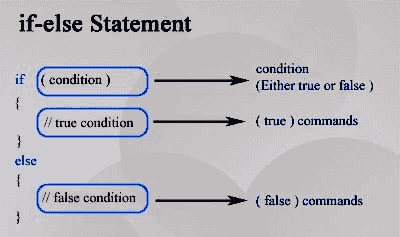top of page
Search

# Detailed Explanation of if, if-else & Nested if-else Conditions with Examples of C Programming

Decision making is required when one or more conditions are to be tested in a program. If the condition is true then a statement or group of statements will be executed and if the condition is false then another set of statements or statements will be executed.

Shown below is the general form of a typical decision-making structure.

## if Statement

The flow chart of if statement is given aside:## if-else Statement

In C language, the if-else statement is used for decision making.

Syntax:if (expression)

{

statement(s);

}

else

{

statement(s);

}

The expression after the 'if' is called the condition of the if statement. If the expression is evaluated to true, the statement(s) following 'if' clause execute and if the expression evaluates to a false value, the statement following the 'else' clause executes. See the following pictorial presentation of the if-else statement.

Examples:

1. A program to find the largest of 2 numbers: -

#include <stdio.h>

int main()

{

int x, y;

printf("Enter 2 different integers");

scanf("%d %d",&x,&y);

if(x>y) printf("The largest of the 2 numbers is %d",x);

else printf("The largest of the 2 numbers is %d",y);

return(0);

}

2. A program to check whether 2 numbers are equal: -

#include <stdio.h>

int main()

{

int x,y;

printf("Enter 2 integers");

scanf("%d %d",&x,&y);

if(x==y) printf("The numbers are equal");

else printf("The numbers are not equal");

return(0);

}

3. A program to find whether a person is eligible to vote:-

#include <stdio.h>

int main()

{

float age;

printf("Enter the age of the person:");

scanf("%f",&age);

if(age>=18) printf("Eligible to vote");

else printf("Not eligible to vote");

return(0);

}

## Nested if...else statement (if...else if....else Statement)

Syntax:

if (expression1)

{

statement(s);

}

else if (expression2)

{

statement(s);

}

·

·

·

else if (expression n)

{

statement(s);

}

else

{

statements;

}

At the time of execution, the compiler will check the expression (condition) one after the other, if any expression (condition) is found to be true, the corresponding statement will be executed. If none of the expression (condition) is true then the statement after the last else will be executed.

Examples:

1. A program to find the largest of 2 numbers or if they are equal: -

#include <stdio.h>

int main()

{

int x,y;

printf("Enter 2 integers");

scanf("%d %d",&x,&y);

if(x==y) printf("The numbers are equal");

else if(x>y)

printf("The largest of the 2 numbers is %d",x);

else

printf("The largest of the 2 numbers is %d",y);

return(0);

}

2. A program to find the grades obtained:

# include<stdio.h>

int main()

{

float mark;

printf ("Enter the marks out of 100: ");

scanf("%f",&mark);

if(mark>90)

else if (mark<=90 && mark > 80)

else if (mark<=80 && mark > 70)

else if (mark<=70 && mark > 60)

else if (mark<=60 && mark > 50)

else if (mark<=50 && mark > 40)

else if (mark<=40 && mark > 32)